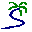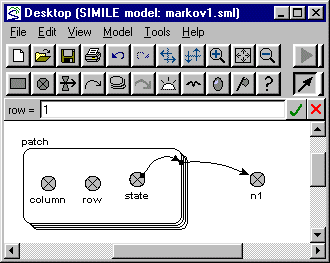# Model entry

Elementary two-state Markov model of land-use dynamics - Model catalogue - Simulistics.com

 Search Simulistics Model catalogue Listed by keyword Listed by ID Listed by title Listed by date added

# Elementary two-state Markov model of land-use dynamics

Model : markov1
Simile version : 3.1+
Keywords : Land-use change ; Markov model ;

## Description

Space is represented as a square grid. Each cell can be in one of two states (0 or 1). There is a probability p1 that a cell in state 0 will change to state 1, and a probability p2 that a cell in state 1 will change to state 0: p1 and p2 are constant. There is no interaction between neighbouring cells.

A practical handout, in pdf format, for using this and two other models of land-use dynamics is available here

## Files

### Model file

Click on the icon to download the model file. (You will need Simile to examine and run the model. A free evaluation version is available from the products page.)

Some browsers may attempt to display the model file, rather than open it in Simile; in this case, use the browser back button to return to this page, and use the context menu (invoked by right-clicking on the link) to save the target file to disk.markov1.sml

## Diagram## Equations

```Equations in ../Desktop
n1 = sum([state])
where: [state] = patch/state

Equations in patch
state = (if time(1) == 0 then 0
elseif prev(1) == 0 and rand_var(0,1)<0.1 then 1
elseif prev(1) == 1 and rand_var(0,1)<0.1 then 0
else prev(1))
column = fmod(index(1)-1,10)+1
row = 1
```